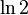# Learning curve

## Definition

The term learning curve is typically used in the context of a graph where the axes are as follows:

• The horizontal axis represents a hyperparameter. This could be a model hyperparameter (a hyperparameter controlling the choice of model), regularization hyperparameter (a hyperparameter controlling how we regularize), or learning algorithm hyperparameter (a hyperparameter controlling how the learning algorithm proceeds).
• The vertical axis represents the cost function value. There are several different cost function values that could be plotted:
• The value of the regularized cost function on the training set (this is the function that we are ostensibly trying to optimize with the learning algorithm).
• The value of the unregularized cost function on the training set.
• The value of the unregularized cost function on the cross-validation set (or test set).

In many cases, we plot all the curves together in the same picture, so that we can compare the training and test errors.

When we plot the learning curve with respect to a particular hyperparameter, we are either holding everything else fixed or choosing all other ways in a manner that they are optimal for the choice of hyperparameter (i.e., multi-staged optimization).

## General concepts

We say that a cost function value is "high" if it is similar to or more than the cost function value one could get without any knowledge of the training data. For instance, in the logistic regression problem with a logarithmic cost function, always predicting a probability of 0.5 yields an error of$\ln 2$ on all data sets, so a cost function that is close to, or greater than,$\ln 2$, is high.

We say that a cost function value is "low" if it is close to zero.

• We say that a particular hyperparameter value exhibits high bias if the training error and cross-validation error are both high, we may also say that the model is underfitted.
• We say that a particular hyperparameter value exhibits high variance if the training error is low but the cross-validation error is high. We also call this a situation of overfitting.

The interpretation of the high bias and high variance situations depends on what sort of hyperparameter our model is in terms of. Some cases are discussed below.

Type of hyperparameter Meaning of high bias case Meaning of high variance case (overfitting)
model hyperparameter The model isn't sufficiently powerful to predict the output. We might need to consider a more powerful model, or add more derived features (in the case of a polynomial model where the hyperparameter is the degree of the polynomial, consider increasing the degree of the polynomial). The model has too many parameters. Consider switching to a simpler model, or reducing the number of derived features (in the case of a polynomial model, consider reducing the degree of the polynomial)
regularization hyperparameter The regularization is too strong. Consider reducing the regularization of the model, to allow for more complicated sets of parameter values. The regularization is too weak. Consider increasing the regularization of the model, to enforce simplicity and reduce overfitting.
learning algorithm hyperparameter The learning algorithm is not being run well enough (for instance, the number of iterations is too little, or the learning rate is too far from optimal). Consider running the algorithm longer or tweaking the learning rate to force faster convergence. The learning algorithm is being run too long, or with too good a learning rate, and as a result it is learning the noise in the training set rather than the generalizable aspects. Consider early stopping (reducing the number of iterations).